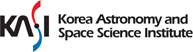### Orbit Analysis and Estimation

### Astrometry

Astrometry is the oldest branch of Astronomy. It is still the most applicable and fundamental. For many years, the position and movement of celestial bodies have been determined with the highest precision (Astrometry) in order to develop and maintain a system of time and a reference coordinate system. Astrometry research gave birth to the concept of time and almanacs and made major contributions to the study of modern cosmology, as well as celestial mechanics and dynamics. In terms of space situational awareness, astrometry is mainly used to analyze observational data and to determine the precise positions of artificial and natural space objects.

#### Subjects

• Astronomical time system: Along with the atomic time system, astrometry still defines the coordinated Universal Time by the precise observation of the movements of the celestial bodies.
• Reference system: From the positional observation of the pre-selected quasar and the star, we can determine the ICRS and ITRS to ICRF and ITRF respectively.
• Proper motion
• Earth rotation and revolution
• Dynamical evolution of the Solar system
• Positional astronomy

### Celestial Mechanics

Celestial mechanics is the study of the properties of motion of natural and manmade space objects. This includes their coordinates, trajectory, orbit propagation, orbit determination, and orbit estimation, including the theory of errors in the definition of outer space. After the law of gravity and the law of the elliptical motion were proposed by Newton and Kepler, respectively, most people, including many considered to be experts in the fields of physics and mathematics, thought that we knew everything that could be known about celestial mechanics. This state of affairs continued until the early 20th century, but when the space race began in the middle of the 20th century, scientists once again began studying celestial mechanics and many studies were produced. Now, celestial mechanics is an important area of study where orbit propagation of artificial and natural space objects, dynamics models, perturbation analysis, orbit determination and various other theories related to Celestial Mechanics are applied directly to understand what is happening in space.

#### Subjects

• Geocentric and heliocentric dynamics
• Classical orbit elements and various type of orbit elements
• General perturbation theory
• Special perturbation theory
• Lagrange planetary equation
• Kepler equation
• Encke propagation method of orbit
• Cowell propagation method of orbit
• Hill’s equation
• Bracket theory
• Gauss, Laplace, Gooding method of orbit determination
• Orbit estimation by Differential Correction

### Orbit estimation

This is a statistical method for estimating the value of the the orbital, geophysical and space environmental parameters using the observed data and experience with a random element. Additionally, linearized dynamical characteristics of the satellites and asteroids are used, along with observation models, to estimate the final orbit. This linearized estimation method is used to determine the final orbit of the observed space objects, to analyze the probability of collisions between space objects, and to estimate the space safety risk. Additionally, by using precise orbit determinations a number of geophysical parameters can be calculated.

#### Subjects

• Batch type filter
• Sequential type filter
• Precise orbit estimation of low Earth orbit satellites
• Geo potential field with the SLR observation of several geodesy satellites
• Atmosphere profile
• Parameter estimation like SRP, ERP
• Lunar orbit analysis
• Determination of ITRF with CRS GPS observation
• Tide (ocean, solid Earth) parameter determination